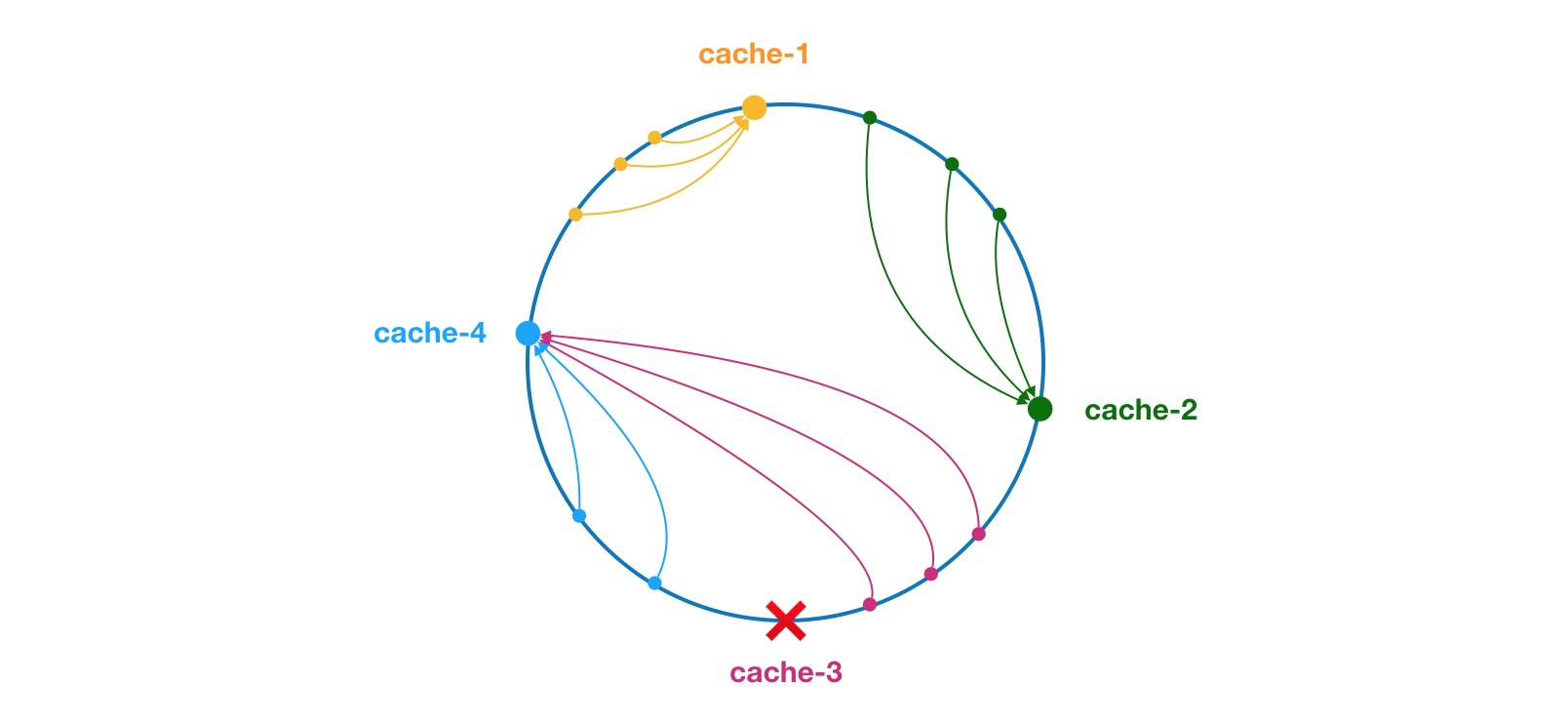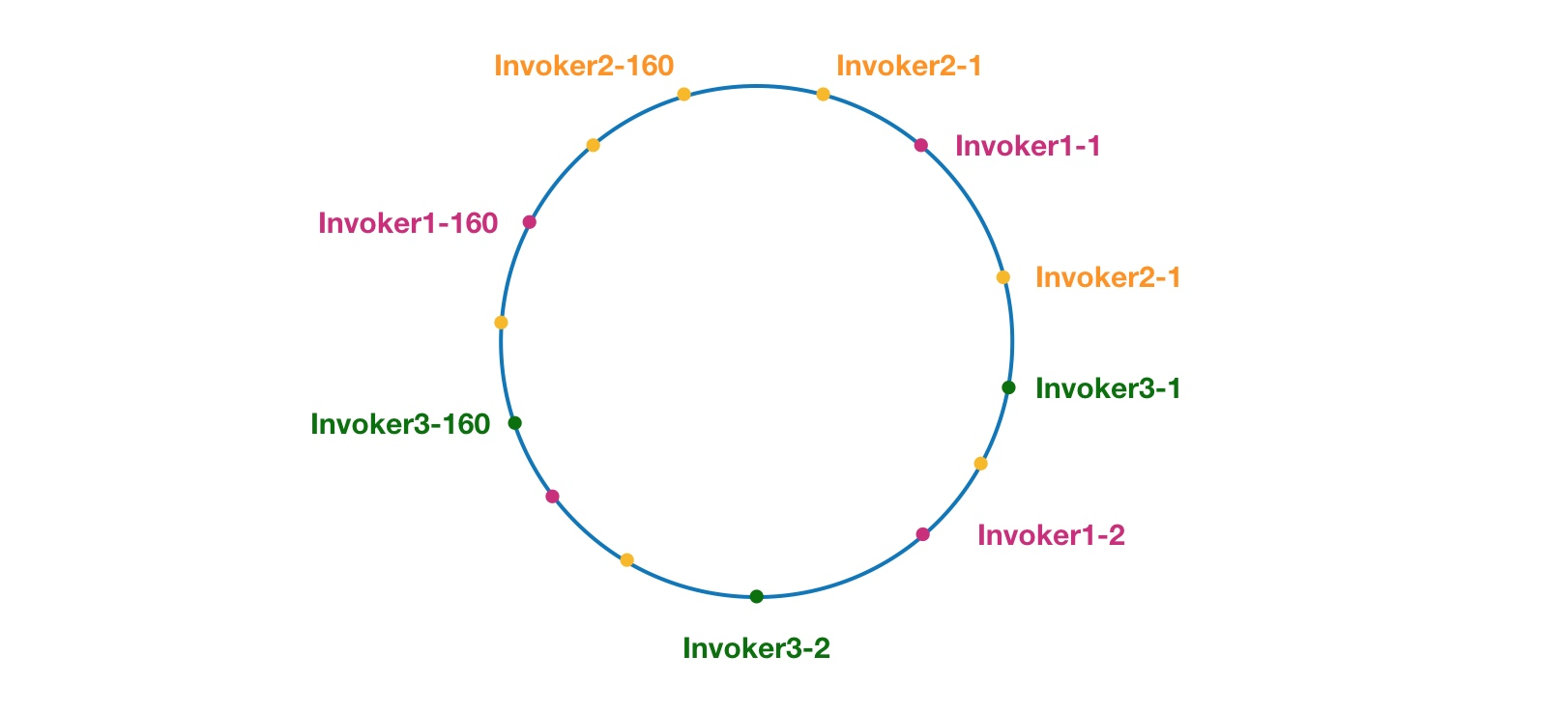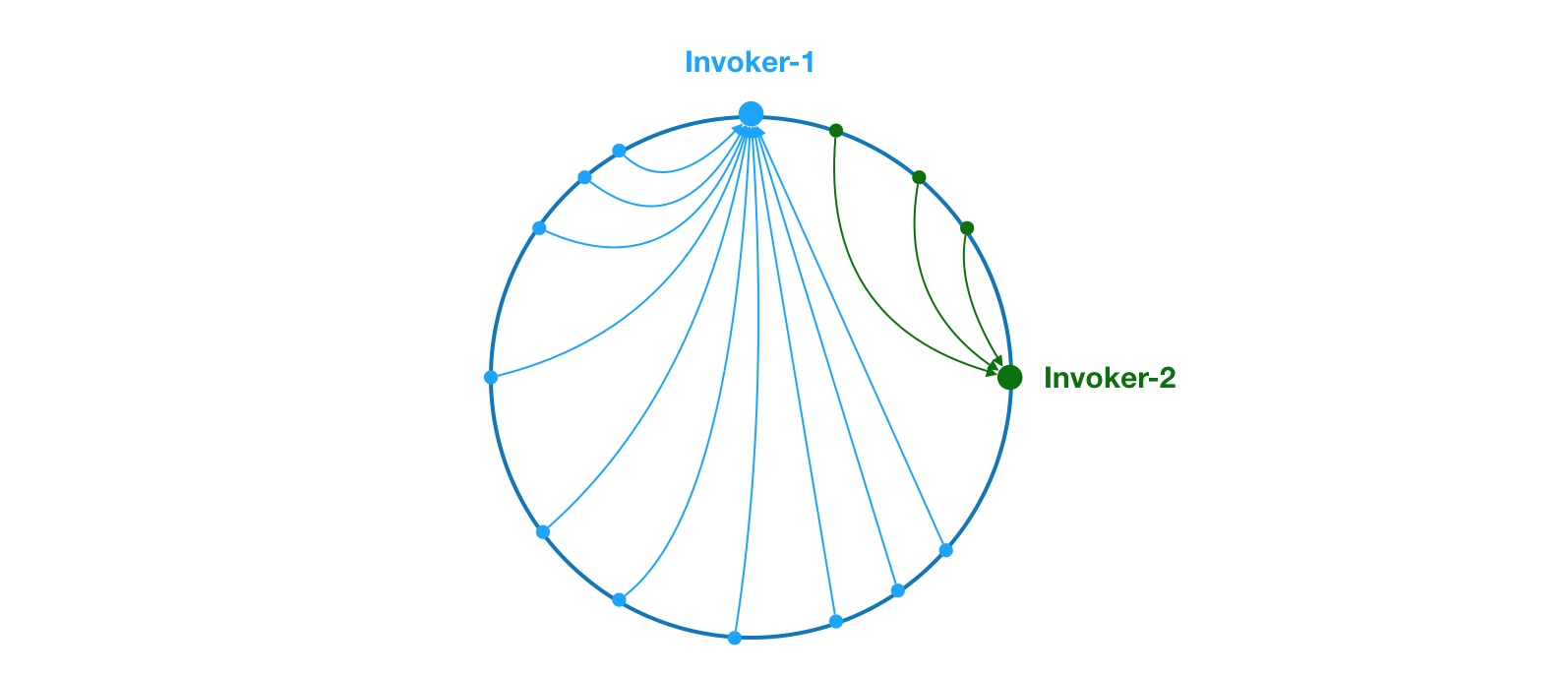# 负载均衡

## 2.源码分析

``````@Override
public <T> Invoker<T> select(List<Invoker<T>> invokers, URL url, Invocation invocation) {
if (invokers == null || invokers.isEmpty())
return null;
// 如果 invokers 列表中仅有一个 Invoker，直接返回即可，无需进行负载均衡
if (invokers.size() == 1)
return invokers.get(0);

// 调用 doSelect 方法进行负载均衡，该方法为抽象方法，由子类实现
return doSelect(invokers, url, invocation);
}

protected abstract <T> Invoker<T> doSelect(List<Invoker<T>> invokers, URL url, Invocation invocation);
``````

select 方法的逻辑比较简单，首先会检测 invokers 集合的合法性，然后再检测 invokers 集合元素数量。如果只包含一个 Invoker，直接返回该 Inovker 即可。如果包含多个 Invoker，此时需要通过负载均衡算法选择一个 Invoker。具体的负载均衡算法由子类实现，接下来章节会对这些子类一一进行详细分析。

``````protected int getWeight(Invoker<?> invoker, Invocation invocation) {
// 从 url 中获取权重 weight 配置值
int weight = invoker.getUrl().getMethodParameter(invocation.getMethodName(), Constants.WEIGHT_KEY, Constants.DEFAULT_WEIGHT);
if (weight > 0) {
// 获取服务提供者启动时间戳
long timestamp = invoker.getUrl().getParameter(Constants.REMOTE_TIMESTAMP_KEY, 0L);
if (timestamp > 0L) {
// 计算服务提供者运行时长
int uptime = (int) (System.currentTimeMillis() - timestamp);
// 获取服务预热时间，默认为10分钟
int warmup = invoker.getUrl().getParameter(Constants.WARMUP_KEY, Constants.DEFAULT_WARMUP);
// 如果服务运行时间小于预热时间，则重新计算服务权重，即降权
if (uptime > 0 && uptime < warmup) {
// 重新计算服务权重
weight = calculateWarmupWeight(uptime, warmup, weight);
}
}
}
return weight;
}

static int calculateWarmupWeight(int uptime, int warmup, int weight) {
// 计算权重，下面代码逻辑上形似于 (uptime / warmup) * weight。
// 随着服务运行时间 uptime 增大，权重计算值 ww 会慢慢接近配置值 weight
int ww = (int) ((float) uptime / ((float) warmup / (float) weight));
return ww < 1 ? 1 : (ww > weight ? weight : ww);
}
``````

RandomLoadBalance 是加权随机算法的具体实现，它的算法思想很简单。假设我们有一组服务器 servers = [A, B, C]，他们对应的权重为 weights = [5, 3, 2]，权重总和为10。现在把这些权重值平铺在一维坐标值上，[0, 5) 区间属于服务器 A，[5, 8) 区间属于服务器 B，[8, 10) 区间属于服务器 C。接下来通过随机数生成器生成一个范围在 [0, 10) 之间的随机数，然后计算这个随机数会落到哪个区间上。比如数字3会落到服务器 A 对应的区间上，此时返回服务器 A 即可。权重越大的机器，在坐标轴上对应的区间范围就越大，因此随机数生成器生成的数字就会有更大的概率落到此区间内。只要随机数生成器产生的随机数分布性很好，在经过多次选择后，每个服务器被选中的次数比例接近其权重比例。比如，经过一万次选择后，服务器 A 被选中的次数大约为5000次，服务器 B 被选中的次数约为3000次，服务器 C 被选中的次数约为2000次。

``````public class RandomLoadBalance extends AbstractLoadBalance {

public static final String NAME = "random";

private final Random random = new Random();

@Override
protected <T> Invoker<T> doSelect(List<Invoker<T>> invokers, URL url, Invocation invocation) {
int length = invokers.size();
int totalWeight = 0;
boolean sameWeight = true;
// 下面这个循环有两个作用，第一是计算总权重 totalWeight，
// 第二是检测每个服务提供者的权重是否相同
for (int i = 0; i < length; i++) {
int weight = getWeight(invokers.get(i), invocation);
// 累加权重
totalWeight += weight;
// 检测当前服务提供者的权重与上一个服务提供者的权重是否相同，
// 不相同的话，则将 sameWeight 置为 false。
if (sameWeight && i > 0
&& weight != getWeight(invokers.get(i - 1), invocation)) {
sameWeight = false;
}
}

// 下面的 if 分支主要用于获取随机数，并计算随机数落在哪个区间上
if (totalWeight > 0 && !sameWeight) {
// 随机获取一个 [0, totalWeight) 区间内的数字
int offset = random.nextInt(totalWeight);
// 循环让 offset 数减去服务提供者权重值，当 offset 小于0时，返回相应的 Invoker。
// 举例说明一下，我们有 servers = [A, B, C]，weights = [5, 3, 2]，offset = 7。
// 第一次循环，offset - 5 = 2 > 0，即 offset > 5，
// 表明其不会落在服务器 A 对应的区间上。
// 第二次循环，offset - 3 = -1 < 0，即 5 < offset < 8，
// 表明其会落在服务器 B 对应的区间上
for (int i = 0; i < length; i++) {
// 让随机值 offset 减去权重值
offset -= getWeight(invokers.get(i), invocation);
if (offset < 0) {
// 返回相应的 Invoker
return invokers.get(i);
}
}
}

// 如果所有服务提供者权重值相同，此时直接随机返回一个即可
return invokers.get(random.nextInt(length));
}
}
``````

``````public class LeastActiveLoadBalance extends AbstractLoadBalance {

public static final String NAME = "leastactive";

private final Random random = new Random();

@Override
protected <T> Invoker<T> doSelect(List<Invoker<T>> invokers, URL url, Invocation invocation) {
int length = invokers.size();
// 最小的活跃数
int leastActive = -1;
// 具有相同“最小活跃数”的服务者提供者（以下用 Invoker 代称）数量
int leastCount = 0;
// leastIndexs 用于记录具有相同“最小活跃数”的 Invoker 在 invokers 列表中的下标信息
int[] leastIndexs = new int[length];
int totalWeight = 0;
// 第一个最小活跃数的 Invoker 权重值，用于与其他具有相同最小活跃数的 Invoker 的权重进行对比，
// 以检测是否“所有具有相同最小活跃数的 Invoker 的权重”均相等
int firstWeight = 0;
boolean sameWeight = true;

// 遍历 invokers 列表
for (int i = 0; i < length; i++) {
Invoker<T> invoker = invokers.get(i);
// 获取 Invoker 对应的活跃数
int active = RpcStatus.getStatus(invoker.getUrl(), invocation.getMethodName()).getActive();
// 获取权重 - ⭐️
int weight = invoker.getUrl().getMethodParameter(invocation.getMethodName(), Constants.WEIGHT_KEY, Constants.DEFAULT_WEIGHT);
// 发现更小的活跃数，重新开始
if (leastActive == -1 || active < leastActive) {
// 使用当前活跃数 active 更新最小活跃数 leastActive
leastActive = active;
// 更新 leastCount 为 1
leastCount = 1;
// 记录当前下标值到 leastIndexs 中
leastIndexs = i;
totalWeight = weight;
firstWeight = weight;
sameWeight = true;

// 当前 Invoker 的活跃数 active 与最小活跃数 leastActive 相同
} else if (active == leastActive) {
// 在 leastIndexs 中记录下当前 Invoker 在 invokers 集合中的下标
leastIndexs[leastCount++] = i;
// 累加权重
totalWeight += weight;
// 检测当前 Invoker 的权重与 firstWeight 是否相等，
// 不相等则将 sameWeight 置为 false
if (sameWeight && i > 0
&& weight != firstWeight) {
sameWeight = false;
}
}
}

// 当只有一个 Invoker 具有最小活跃数，此时直接返回该 Invoker 即可
if (leastCount == 1) {
return invokers.get(leastIndexs);
}

// 有多个 Invoker 具有相同的最小活跃数，但它们之间的权重不同
if (!sameWeight && totalWeight > 0) {
// 随机生成一个 [0, totalWeight) 之间的数字
int offsetWeight = random.nextInt(totalWeight);
// 循环让随机数减去具有最小活跃数的 Invoker 的权重值，
// 当 offset 小于等于0时，返回相应的 Invoker
for (int i = 0; i < leastCount; i++) {
int leastIndex = leastIndexs[i];
// 获取权重值，并让随机数减去权重值 - ⭐️
offsetWeight -= getWeight(invokers.get(leastIndex), invocation);
if (offsetWeight <= 0)
return invokers.get(leastIndex);
}
}
// 如果权重相同或权重为0时，随机返回一个 Invoker
return invokers.get(leastIndexs[random.nextInt(leastCount)]);
}
}
``````

1. 遍历 invokers 列表，寻找活跃数最小的 Invoker
2. 如果有多个 Invoker 具有相同的最小活跃数，此时记录下这些 Invoker 在 invokers 集合中的下标，并累加它们的权重，比较它们的权重值是否相等
3. 如果只有一个 Invoker 具有最小的活跃数，此时直接返回该 Invoker 即可
4. 如果有多个 Invoker 具有最小活跃数，且它们的权重不相等，此时处理方式和 RandomLoadBalance 一致
5. 如果有多个 Invoker 具有最小活跃数，但它们的权重相等，此时随机返回一个即可

``````int weight = invoker.getUrl().getMethodParameter(invocation.getMethodName(), Constants.WEIGHT_KEY, Constants.DEFAULT_WEIGHT);
``````
``````offsetWeight -= getWeight(invokers.get(leastIndex), invocation);
``````

``````// afterWarmup 等价于上面的 weight 变量，这样命名是为了强调该变量经过了 warmup 降权处理
int afterWarmup = getWeight(invoker, invocation);
``````

``````int offsetWeight = random.nextInt(totalWeight);
for (int i = 0; i < leastCount; i++) {
int leastIndex = leastIndexs[i];
offsetWeight -= getWeight(invokers.get(leastIndex), invocation);
if (offsetWeight <= 0)    // ❌
return invokers.get(leastIndex);
}
``````

``````int offsetWeight = random.nextInt(totalWeight) + 1;
````````````public class ConsistentHashLoadBalance extends AbstractLoadBalance {

private final ConcurrentMap<String, ConsistentHashSelector<?>> selectors =
new ConcurrentHashMap<String, ConsistentHashSelector<?>>();

@Override
protected <T> Invoker<T> doSelect(List<Invoker<T>> invokers, URL url, Invocation invocation) {
String methodName = RpcUtils.getMethodName(invocation);
String key = invokers.get(0).getUrl().getServiceKey() + "." + methodName;

// 获取 invokers 原始的 hashcode
int identityHashCode = System.identityHashCode(invokers);
ConsistentHashSelector<T> selector = (ConsistentHashSelector<T>) selectors.get(key);
// 如果 invokers 是一个新的 List 对象，意味着服务提供者数量发生了变化，可能新增也可能减少了。
// 此时 selector.identityHashCode != identityHashCode 条件成立
if (selector == null || selector.identityHashCode != identityHashCode) {
// 创建新的 ConsistentHashSelector
selectors.put(key, new ConsistentHashSelector<T>(invokers, methodName, identityHashCode));
selector = (ConsistentHashSelector<T>) selectors.get(key);
}

// 调用 ConsistentHashSelector 的 select 方法选择 Invoker
return selector.select(invocation);
}

private static final class ConsistentHashSelector<T> {...}
}
``````

``````private static final class ConsistentHashSelector<T> {

// 使用 TreeMap 存储 Invoker 虚拟节点
private final TreeMap<Long, Invoker<T>> virtualInvokers;

private final int replicaNumber;

private final int identityHashCode;

private final int[] argumentIndex;

ConsistentHashSelector(List<Invoker<T>> invokers, String methodName, int identityHashCode) {
this.virtualInvokers = new TreeMap<Long, Invoker<T>>();
this.identityHashCode = identityHashCode;
URL url = invokers.get(0).getUrl();
// 获取虚拟节点数，默认为160
this.replicaNumber = url.getMethodParameter(methodName, "hash.nodes", 160);
// 获取参与 hash 计算的参数下标值，默认对第一个参数进行 hash 运算
String[] index = Constants.COMMA_SPLIT_PATTERN.split(url.getMethodParameter(methodName, "hash.arguments", "0"));
argumentIndex = new int[index.length];
for (int i = 0; i < index.length; i++) {
argumentIndex[i] = Integer.parseInt(index[i]);
}
for (Invoker<T> invoker : invokers) {
for (int i = 0; i < replicaNumber / 4; i++) {
// 对 address + i 进行 md5 运算，得到一个长度为16的字节数组
byte[] digest = md5(address + i);
// 对 digest 部分字节进行4次 hash 运算，得到四个不同的 long 型正整数
for (int h = 0; h < 4; h++) {
// h = 0 时，取 digest 中下标为 0 ~ 3 的4个字节进行位运算
// h = 1 时，取 digest 中下标为 4 ~ 7 的4个字节进行位运算
// h = 2, h = 3 时过程同上
long m = hash(digest, h);
// 将 hash 到 invoker 的映射关系存储到 virtualInvokers 中，
// virtualInvokers 需要提供高效的查询操作，因此选用 TreeMap 作为存储结构
virtualInvokers.put(m, invoker);
}
}
}
}
}
``````

``````public Invoker<T> select(Invocation invocation) {
// 将参数转为 key
String key = toKey(invocation.getArguments());
// 对参数 key 进行 md5 运算
byte[] digest = md5(key);
// 取 digest 数组的前四个字节进行 hash 运算，再将 hash 值传给 selectForKey 方法，
// 寻找合适的 Invoker
return selectForKey(hash(digest, 0));
}

private Invoker<T> selectForKey(long hash) {
// 到 TreeMap 中查找第一个节点值大于或等于当前 hash 的 Invoker
Map.Entry<Long, Invoker<T>> entry = virtualInvokers.tailMap(hash, true).firstEntry();
// 如果 hash 大于 Invoker 在圆环上最大的位置，此时 entry = null，
// 需要将 TreeMap 的头节点赋值给 entry
if (entry == null) {
entry = virtualInvokers.firstEntry();
}

// 返回 Invoker
return entry.getValue();
}
``````

``````public class RoundRobinLoadBalance extends AbstractLoadBalance {

public static final String NAME = "roundrobin";

private final ConcurrentMap<String, AtomicPositiveInteger> sequences =
new ConcurrentHashMap<String, AtomicPositiveInteger>();

@Override
protected <T> Invoker<T> doSelect(List<Invoker<T>> invokers, URL url, Invocation invocation) {
// key = 全限定类名 + "." + 方法名，比如 com.xxx.DemoService.sayHello
String key = invokers.get(0).getUrl().getServiceKey() + "." + invocation.getMethodName();
int length = invokers.size();
// 最大权重
int maxWeight = 0;
// 最小权重
int minWeight = Integer.MAX_VALUE;
// 权重总和
int weightSum = 0;

// 下面这个循环主要用于查找最大和最小权重，计算权重总和等
for (int i = 0; i < length; i++) {
int weight = getWeight(invokers.get(i), invocation);
// 获取最大和最小权重
maxWeight = Math.max(maxWeight, weight);
minWeight = Math.min(minWeight, weight);
if (weight > 0) {
// 将 weight 封装到 IntegerWrapper 中
invokerToWeightMap.put(invokers.get(i), new IntegerWrapper(weight));
// 累加权重
weightSum += weight;
}
}

// 查找 key 对应的对应 AtomicPositiveInteger 实例，为空则创建。
// 这里可以把 AtomicPositiveInteger 看成一个黑盒，大家只要知道
// AtomicPositiveInteger 用于记录服务的调用编号即可。至于细节，
// 大家如果感兴趣，可以自行分析
AtomicPositiveInteger sequence = sequences.get(key);
if (sequence == null) {
sequences.putIfAbsent(key, new AtomicPositiveInteger());
sequence = sequences.get(key);
}

// 获取当前的调用编号
int currentSequence = sequence.getAndIncrement();
// 如果最小权重小于最大权重，表明服务提供者之间的权重是不相等的
if (maxWeight > 0 && minWeight < maxWeight) {
// 使用调用编号对权重总和进行取余操作
int mod = currentSequence % weightSum;
// 进行 maxWeight 次遍历
for (int i = 0; i < maxWeight; i++) {
// 遍历 invokerToWeightMap
for (Map.Entry<Invoker<T>, IntegerWrapper> each : invokerToWeightMap.entrySet()) {
// 获取 Invoker
final Invoker<T> k = each.getKey();
// 获取权重包装类 IntegerWrapper
final IntegerWrapper v = each.getValue();

// 如果 mod = 0，且权重大于0，此时返回相应的 Invoker
if (mod == 0 && v.getValue() > 0) {
return k;
}

// mod != 0，且权重大于0，此时对权重和 mod 分别进行自减操作
if (v.getValue() > 0) {
v.decrement();
mod--;
}
}
}
}

// 服务提供者之间的权重相等，此时通过轮询选择 Invoker
return invokers.get(currentSequence % length);
}

// IntegerWrapper 是一个 int 包装类，主要包含了一个自减方法。
private static final class IntegerWrapper {
private int value;

public void decrement() {
this.value--;
}

// 省略部分代码
}
}
``````

mod = 0：满足条件，此时直接返回服务器 A

mod = 1：需要进行一次递减操作才能满足条件，此时返回服务器 B

mod = 2：需要进行两次递减操作才能满足条件，此时返回服务器 C

mod = 3：需要进行三次递减操作才能满足条件，经过递减后，服务器权重为 [1, 4, 0]，此时返回服务器 A

mod = 4：需要进行四次递减操作才能满足条件，经过递减后，服务器权重为 [0, 4, 0]，此时返回服务器 B

mod = 5：需要进行五次递减操作才能满足条件，经过递减后，服务器权重为 [0, 3, 0]，此时返回服务器 B

mod = 6：需要进行六次递减操作才能满足条件，经过递减后，服务器权重为 [0, 2, 0]，此时返回服务器 B

mod = 7：需要进行七次递减操作才能满足条件，经过递减后，服务器权重为 [0, 1, 0]，此时返回服务器 B

``````public class RoundRobinLoadBalance extends AbstractLoadBalance {

public static final String NAME = "roundrobin";

private final ConcurrentMap<String, AtomicPositiveInteger> sequences = new ConcurrentHashMap<String, AtomicPositiveInteger>();

private final ConcurrentMap<String, AtomicPositiveInteger> indexSeqs = new ConcurrentHashMap<String, AtomicPositiveInteger>();

@Override
protected <T> Invoker<T> doSelect(List<Invoker<T>> invokers, URL url, Invocation invocation) {
String key = invokers.get(0).getUrl().getServiceKey() + "." + invocation.getMethodName();
int length = invokers.size();
int maxWeight = 0;
int minWeight = Integer.MAX_VALUE;
final List<Invoker<T>> invokerToWeightList = new ArrayList<>();

// 查找最大和最小权重
for (int i = 0; i < length; i++) {
int weight = getWeight(invokers.get(i), invocation);
maxWeight = Math.max(maxWeight, weight);
minWeight = Math.min(minWeight, weight);
if (weight > 0) {
}
}

// 获取当前服务对应的调用序列对象 AtomicPositiveInteger
AtomicPositiveInteger sequence = sequences.get(key);
if (sequence == null) {
// 创建 AtomicPositiveInteger，默认值为0
sequences.putIfAbsent(key, new AtomicPositiveInteger());
sequence = sequences.get(key);
}

// 获取下标序列对象 AtomicPositiveInteger
AtomicPositiveInteger indexSeq = indexSeqs.get(key);
if (indexSeq == null) {
// 创建 AtomicPositiveInteger，默认值为 -1
indexSeqs.putIfAbsent(key, new AtomicPositiveInteger(-1));
indexSeq = indexSeqs.get(key);
}

if (maxWeight > 0 && minWeight < maxWeight) {
length = invokerToWeightList.size();
while (true) {
int index = indexSeq.incrementAndGet() % length;
int currentWeight = sequence.get() % maxWeight;

// 每循环一轮（index = 0），重新计算 currentWeight
if (index == 0) {
currentWeight = sequence.incrementAndGet() % maxWeight;
}

// 检测 Invoker 的权重是否大于 currentWeight，大于则返回
if (getWeight(invokerToWeightList.get(index), invocation) > currentWeight) {
return invokerToWeightList.get(index);
}
}
}

// 所有 Invoker 权重相等，此时进行普通的轮询即可
return invokers.get(sequence.incrementAndGet() % length);
}
}
``````

1[5, 1, 1]A[-2, 1, 1]
2[3, 2, 2]A[-4, 2, 2]
3[1, 3, 3]B[1, -4, 3]
4[6, -3, 4]A[-1, -3, 4]
5[4, -2, 5]C[4, -2, -2]
6[9, -1, -1]A[2, -1, -1]
7[7, 0, 0]A[0, 0, 0]

``````public class RoundRobinLoadBalance extends AbstractLoadBalance {
public static final String NAME = "roundrobin";

private static int RECYCLE_PERIOD = 60000;

protected static class WeightedRoundRobin {
// 服务提供者权重
private int weight;
// 当前权重
private AtomicLong current = new AtomicLong(0);
// 最后一次更新时间
private long lastUpdate;

public void setWeight(int weight) {
this.weight = weight;
// 初始情况下，current = 0
current.set(0);
}
public long increaseCurrent() {
// current = current + weight；
}
public void sel(int total) {
// current = current - total;
}
}

// 嵌套 Map 结构，存储的数据结构示例如下：
// {
//     "UserService.query": {
//         "url1": WeightedRoundRobin@123,
//         "url2": WeightedRoundRobin@456,
//     },
//     "UserService.update": {
//         "url1": WeightedRoundRobin@123,
//         "url2": WeightedRoundRobin@456,
//     }
// }
// 最外层为服务类名 + 方法名，第二层为 url 到 WeightedRoundRobin 的映射关系。
// 这里我们可以将 url 看成是服务提供者的 id
private ConcurrentMap<String, ConcurrentMap<String, WeightedRoundRobin>> methodWeightMap = new ConcurrentHashMap<String, ConcurrentMap<String, WeightedRoundRobin>>();

// 原子更新锁
private AtomicBoolean updateLock = new AtomicBoolean();

@Override
protected <T> Invoker<T> doSelect(List<Invoker<T>> invokers, URL url, Invocation invocation) {
String key = invokers.get(0).getUrl().getServiceKey() + "." + invocation.getMethodName();
// 获取 url 到 WeightedRoundRobin 映射表，如果为空，则创建一个新的
ConcurrentMap<String, WeightedRoundRobin> map = methodWeightMap.get(key);
if (map == null) {
methodWeightMap.putIfAbsent(key, new ConcurrentHashMap<String, WeightedRoundRobin>());
map = methodWeightMap.get(key);
}
int totalWeight = 0;
long maxCurrent = Long.MIN_VALUE;

// 获取当前时间
long now = System.currentTimeMillis();
Invoker<T> selectedInvoker = null;
WeightedRoundRobin selectedWRR = null;

// 下面这个循环主要做了这样几件事情：
//   1. 遍历 Invoker 列表，检测当前 Invoker 是否有
//      相应的 WeightedRoundRobin，没有则创建
//   2. 检测 Invoker 权重是否发生了变化，若变化了，
//      则更新 WeightedRoundRobin 的 weight 字段
//   3. 让 current 字段加上自身权重，等价于 current += weight
//   4. 设置 lastUpdate 字段，即 lastUpdate = now
//   5. 寻找具有最大 current 的 Invoker，以及 Invoker 对应的 WeightedRoundRobin，
//      暂存起来，留作后用
//   6. 计算权重总和
for (Invoker<T> invoker : invokers) {
String identifyString = invoker.getUrl().toIdentityString();
WeightedRoundRobin weightedRoundRobin = map.get(identifyString);
int weight = getWeight(invoker, invocation);
if (weight < 0) {
weight = 0;
}

// 检测当前 Invoker 是否有对应的 WeightedRoundRobin，没有则创建
if (weightedRoundRobin == null) {
weightedRoundRobin = new WeightedRoundRobin();
// 设置 Invoker 权重
weightedRoundRobin.setWeight(weight);
// 存储 url 唯一标识 identifyString 到 weightedRoundRobin 的映射关系
map.putIfAbsent(identifyString, weightedRoundRobin);
weightedRoundRobin = map.get(identifyString);
}
// Invoker 权重不等于 WeightedRoundRobin 中保存的权重，说明权重变化了，此时进行更新
if (weight != weightedRoundRobin.getWeight()) {
weightedRoundRobin.setWeight(weight);
}

// 让 current 加上自身权重，等价于 current += weight
long cur = weightedRoundRobin.increaseCurrent();
// 设置 lastUpdate，表示近期更新过
weightedRoundRobin.setLastUpdate(now);
// 找出最大的 current
if (cur > maxCurrent) {
maxCurrent = cur;
// 将具有最大 current 权重的 Invoker 赋值给 selectedInvoker
selectedInvoker = invoker;
// 将 Invoker 对应的 weightedRoundRobin 赋值给 selectedWRR，留作后用
selectedWRR = weightedRoundRobin;
}

// 计算权重总和
totalWeight += weight;
}

// 对 <identifyString, WeightedRoundRobin> 进行检查，过滤掉长时间未被更新的节点。
// 该节点可能挂了，invokers 中不包含该节点，所以该节点的 lastUpdate 长时间无法被更新。
// 若未更新时长超过阈值后，就会被移除掉，默认阈值为60秒。
if (!updateLock.get() && invokers.size() != map.size()) {
if (updateLock.compareAndSet(false, true)) {
try {
ConcurrentMap<String, WeightedRoundRobin> newMap = new ConcurrentHashMap<String, WeightedRoundRobin>();
// 拷贝
newMap.putAll(map);

// 遍历修改，即移除过期记录
Iterator<Entry<String, WeightedRoundRobin>> it = newMap.entrySet().iterator();
while (it.hasNext()) {
Entry<String, WeightedRoundRobin> item = it.next();
if (now - item.getValue().getLastUpdate() > RECYCLE_PERIOD) {
it.remove();
}
}

// 更新引用
methodWeightMap.put(key, newMap);
} finally {
updateLock.set(false);
}
}
}

if (selectedInvoker != null) {
// 让 current 减去权重总和，等价于 current -= totalWeight
selectedWRR.sel(totalWeight);
// 返回具有最大 current 的 Invoker
return selectedInvoker;
}

// should not happen here
return invokers.get(0);
}
}
``````